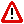To Return to the Prior Page "Click" the Browser Back Button Until the Desired Page Appears

 Natural function, Psi, ΨThe Natural function refers to a mathematical expression.  The Natural function is expressed as: the square of "x" minus "x."  It is the Natural function that interrelates the ordinal numbers zero and plus one, which relates the negative and positive scales of integers.  That is:  2 = -1; 3 = -2; 4 = -3 ...  And of course, +1 = 0, both of which represent the singularity, Infinity.  Each pair of integers, so defined, are referred to as Natural pairs and are, respectively, equal distances from plus one and zero on the number scale.

T
he Natural function is: x2 - x, is referred to as Psi, Ψ.

The Natural function,
Psi, Ψ. is so named, "Natural," because it is the second term of a Brunardot Ellipse Pulser, which is the term of a Pulser that refers to the soliton, "s," of an ellipse.  It is the soliton, in Nature, that underlies all the internal, hyper-relativistic, oscillating, nonlinear structure of Light and mass.  In fact, the soliton is the crux of all the structures of Reality including "space."

When the second term of a Pulser is the Natural function,
Psi, Ψ, of the first term, the subsequent unending, additive, series is referred to as the Brunardot Series
.

 E-mail :  Brunardot@Brunardot.comTerms of:  ©Copyright 1999-2015 by Brunardot

000101 0:01a